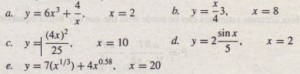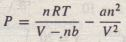Answers to problems marked with an asterisk are given at the end of the text.

1. Make sure you know how to start and quit a MATLAB session. Use MATLAB to make the following calculations, using the values: x = 10, Y = 3. Check the results using a calculator.
a. u = x + Y                b. v = x y              c. w = x  /  y
d. z = sin x                 e. r = 8 sin y        f  s = S sin ( 2 y )
2. Suppose that x = 2 and y = S. Use MATLAB to compute the following.3. Suppose that x = 3 and y = 4. Use MATLAB to compute the following, and check the results with a calculator.4. Evaluate the following expressions in MATLAB for the given value of x.5. Assuming that the variables a, b, c, d, and f are scalars, write MATLAB statements to compute and display the following expressions. Test your statements for the values a = 1.12, b = 2.34, c =0.72, d = 0.81, f = 19.83.6. Use MATLAB to calculate7. The volume of a sphere is given by V =4πr³ r3 /3, where r³/s the radius. Use MATLAB to compute the radius of a sphere having a volume 30 percent greater than that of a sphere of radius 5 ft.
8. *Suppose that x = -7 – 5i and y = 4 + 3i. Use MATLAB to compute
a. x + Y                 b. x y                   c. x / Y
9. Use MATLAB to compute the following. Check your answers by hand.10. Evaluate the following expressions in MATLAB, for the values x =5 +8i, y = -6 +7i. Check your answers by hand.11. Engineers often need to estimate the pressure exerted by a gas in a container. The ideal gas law provides one way of making the estimate. The law isMore accurate estimates can be made with the van der Waals equation:where the term lib is a correction for the volume of the molecules, and the term’4112/V2 is a correction for molecular attractions. The values of a and b depend on the type of gas. The gas constant is R, the absolute temperature , the gas volume is V, and the number of gas molecules is indicated by 11.If Il = I mol of an ideal gas were confined to a volume of V = 22.41 L at O°C(273.2 K), it would exert a pressure of 1 atmosphere. In these units, R = 0.08206.
For chlorine (Ch), a = 6.49 and b = 0.0562. Compare the pressure estimates given by the ideal gas law and the van der Waals equation for 1 mol of Cl² in 22.41 L at 273.2 K. What is the main cause of the difference in the two pressure estimates: the molecular volume or the molecular attractions?
12. The ideal gas law relates thepressure P, volume V, absolute  temperature T, and amount of gas n. The law iswhere R is the gas constant.
An engineer must design a large natural gas storage tank to be expandable to maintain the pressure constant at 2.2 atmospheres. In December when the temperature is 4°F (-15°q, the volume of gas in the tank is 28,500 ft3. What will the volume of the same quantity of gas be in July when the temperature is 88cF (31°C)? (Hint: Use the fact that n, R, and P are constant in this problem. Note also that K =°C +273.2.)
13. Suppose x takes on the values x = I, 1.2, 1.4, … 5. Use MATLAB to compute the array y that results from the function y = 7 sin(4x). Use MATLAB to determine how many elements are in the array y, and the value of the third element in the array y.
14. Use MATLAB to determine how many elements are in the array [sin (-pi /2) : O. 05: cas (0) ]. Use MATLAB to determine the 10th element.
15. Use MATLAB to calculate16. Use MATLAB to calculate.17. The Richter scale is a measure of the intensity of an earthquake. The energy E (in joules) released by the quake is related to the magnitude M on the Richter scale as follows.How much more energy is released by a magnitude 7.3 quake than a 5.5 quake?
18. *Use MATLAB to find the roots of 13×3 + 182×2 – 184x + 2503 = O.
19. Use MATLAB to find the roots of the polynomial 36×3 + 12×2 – 5x + 10.
20. Determine which search path MAT~AB uses on your computer. If you use a lab computer as well as a home computer, compare the two search paths. Where will MATLAB look for a user-created M-file on each computer?
21. Use MATLAB to plot the function T = 6 ln t- 7e over the interval 1 ≤ t ≤ 3: Put a title on the plot and properly label the axes. The variable T represents temperature in degrees Celsius; the variable t represents time in minutes.
22. Use MATLAB to plot the functions u = 2 log (60x + 1) and v=3 cos(6x) over the interval 0 ≤ x ≤ 2. Properly label the plot and each curve. The variables u and v represent speed in miles per hour; the variable x represents distance in miles.
23. The Fourier series is a series representation of a periodic function in terms of sines and cosines. The Fourier series representation of the function
isPlot on the same graph the function I(x) and its series representation using the four terms shown.
24. A cycloid is the curve described by a point P on the circumference of a circular wheel of radius r rolling along the x axis. The curve is described in parametric form by the equations
x = r( Φ – sin Φ)
y = r( 1 – cos Φ)
Use these equations to plot the cycloid for r = 10 inches and 0 ≤ Φ ≤ 4π.
25. Use MATLAB to solve the following set of equations.
7x  + 14y  – 6z = 95
12x – 5y + 9z    = -50
-5x +7y + 15z    = 145
26. It is known that the function y =ax3 +bx2 +ex +d passes through the following (x, y) points: (-1,8), (0,4), (I, 10), and (2, 68). Use the MATLAB left division operator / to compute the coefficients a, b, c, and d by writing and solving four linear equations in terms of the four unknowns a, b, c, and d.
27. Create, save, and run a script file that solves the following set of equations for given values of a, b, and c. Check your file for the case a = 95, b = -50, and c = 145.
7x + 14y – 6z  = a
12x –  5y  + 9z  = b
-5x +  7y  +15z  =c
28. A fence around a field is shaped as shown in Figure.P28. It consists of a rectangle of length L and width W, and a right triangle that is symmetrical about the central horizontal axis of the rectangle. Suppose the width Wis· known (in meters), and the enclosed area A is known (in square meters). Write a MATLAB script file in terms of the given variables Wand A to determine the length L required so that the enclosed area is A. Also determine the total length of fence required. Test your script for the values W = 6 m and A = 80 m2.

29. The four-sided figure shown in Figure P29 consists of two triangles having  a common side a. The law of cosines for the top triangle states thatand a similar equation can be written for the bottom triangle. Develop a procedure for computing the length of side C² if you are given the lengths of sides b¹, b², and C¹, and the angles A¹ and A² in degrees. Write a script file to implement, this procedure. Test your script using the following values; b¹ = 180 m, b² = 165 m, CI = 115 m, AI = 120° and A2 = 100°.

30. A fenced enclosure consists of a rectangle of length L and width 2R, and a semicircle of radius R, as shown in Figure P30. The enclosure is to be built to have an area A of 1600 ft². The cost of the fence is \$40 per foot for the curved portion, and \$30 per foot for the straight sides. Use a plot to determine with a resolution of 0.01 ft the values of Rand L required to minimize the total cost of the fence. Also compute the minimum cost, and use a plot of the cost versus R to analyze the sensitivity of the cost to a ±20 percent change in R from its optimum value.

31. Use the MATLAB help facilities to find information about the following topics and symbols: plot, label, cos, cosine, :, and *.
32. Use the MATLAB help facilities to determine what happens if you use the sqrt function with a negative argument.
33. Use the MATLAB help facilities to determine what happens if you use the exp function with an imaginary argument.
34. Suppose that x [-15,-8,9,8,5) andy [-20,12,-4,8,9).
What is the result of the following operations? Determine the answers by hand, and then use MATLAB to check your answers.
a. z = (x < y)
b. z = (x > y)
c. z = (x -= y)
d. z = (x == y)
e. z = (y > -4)
35. Suppose that x = [-15 , – 8 I 9 , 8 I 5] and y = [ – 20 , 12 , – 4 , 8 , 9 ] .
Use MATLAB to find the values and the indices of the elements in x that are greater than the corresponding elements in y.
36. Write a script file using conditional statements to evaluate the following function. assuming that the scalar variable x has a value. The function is37. Use a for loop to plot the function given in Problem 36 over the interval -2 ≤ x ≤ 6. Properly label the plot. The variable y represents height in kilometers. and the variable x represents time in seconds.
38. Plot the function y = 10(1 – e-x/4) over the interval 0 ≤ x ≤ xmax using a while loop to determine the value of xmax such that y(xmax) = 9.8. Properly label the plot. The variable y represents force in newtons. and the variable x represents time in seconds.
39. Use a for loop to determine the sum of the first 10 terms in the series 5k3 k = 1.2.3.. 10.
40. Use a wh i.Le loop to determine how many terms in the series 2k. k = 1.2. 3… are required for the sum of the terms to exceed 2000. What is the sum for this number of terms?\
41. One bank pays 5.5 percent annual interest. while a second bank pays 4.5 percent annual interest. Determine how much longer it will take to accumulate at least \$50.000 in the second bank account if you deposit \$1000 initially, and \$1000 at the end of each year.
42. a. With what initial speed must you throw a ball vertically for it to reach a height of 20 ft? The ball weighs lib, How does your answer change if the ball weighs 2 lb?
b. Suppose you wanted to throw a steel bar vertically to a height of 20 ft. The bar weighs 2 lb. How much initial speed must the bar have to reach this height? Discuss how the length of the bar affects your answer.
43. Consider the otion of the piston discussed in Example 1.7-1. The piston stroke is the total distance moved by the piston as the crank angle varies from 0° to 1800
a. How does the piston stroke depend on L I and L2?
b. Suppose L2 = 0.5 ft. Use MATLAB to plot the piston motion versus crank angle for- two cases: L 1 = 0.6 ft and L 1 =.1.4 ft. Compare each plot with the plot shown in Figure 1.7-3. Discuss how the shape of the plot depends on the value of L1